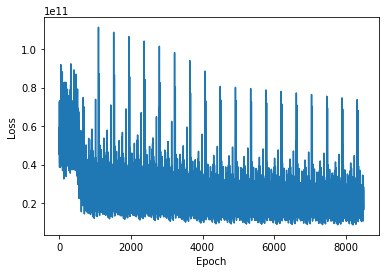# Regression accuracy for house price prediction is too high and not improving

Could somebody help me. I am new to Pytorch and am trying to predict house prices.
I have been able to batch my dataset and do a train-test-split. Even though I trained my model for many epochs my accuracy isn’t really improving or that good.

I used get an error of 1e10 (using MSE). Is this the best it can get or am I doing something wrong.

My training loop:

``````epochs=10
final_losses=[]
lrs=2
dif=3
for j in range(lrs):
###Backward Propogation-- Define the loss_function,define the optimizer
loss_function=nn.MSELoss()
print("\n\n","-"*12)
print("Lr: ", str(10**(-j-dif)))
print("-"*12)
print("\n")
for i in range(epochs):
for step,(x, y) in enumerate(train):#tqdm
y_pred=model(x)
y_pred=y_pred
loss=loss_function(y_pred,y)
final_losses.append(loss)
loss.backward()
optimizer.step()

print("\nEpoch number: {} and the loss : {}".format(i+1,loss.item()))

``````

Testing loop:

``````#### Prediction In X_test data
d=train
predictions=[]
for x,y in test:
#print(x)!
for step,i in enumerate(range(len(x))):
print("Count: {}".format(step+1))
mse=abs(model(x[i]).tolist()-y[i].tolist())**2
print("Expected:",y[i].tolist()," Predicted: ",model(x[i]).tolist()," Error: {:.2e}".format(mse))
break
``````

My error plot:My predictions:

I am confused why my expected vs predicted values differ so much.